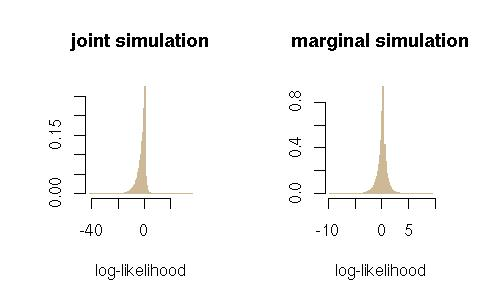Want to share your content on R-bloggers? click here if you have a blog, or here if you don't.At the Edinburgh mixture estimation workshop, Murray Aitkin presented his proposal to compare models via the posterior distribution of the likelihood ratio.$dfrac{L_1(theta_1|x)}{L_2(theta_2|x)}$

As already commented in a post last July, the positive aspect of looking at this quantity rather than at the Bayes factor is that the priors are then allowed to be improper if one simulates from the posteriors for each model, as in Aitkin et al. (2007). My overall feeling has not changed though, namely the ratio should be instead considered under the joint posterior of$(theta_1,theta_2)$, which is [proportional to]$p_1 m_1(x) pi_1(theta_1|x) pi_2(theta_2)+p_2 m_2(x) pi_2(theta_2|x) pi_1(theta_1)$

instead of the product of both posteriors. This of course makes a whole difference, as shown on the next R graph that compares the distribution of the likelihood ratio under the true posterior and under the product of posteriors (when comparing a Poisson model against a negative binomial with$m=5$ successes, when$x=3$). The joint simulation produces a much more supportive argument in favour of the negative binomial model, when compared with the product of the posteriors.Obviously, this joint perspective also cancels the appeal of the approach under improper priors.

Filed under: pictures, R, Statistics, Travel Tagged: Bayesian model choice, Bidean nam Bian, Edinburgh, likelihood ratio, mixtures